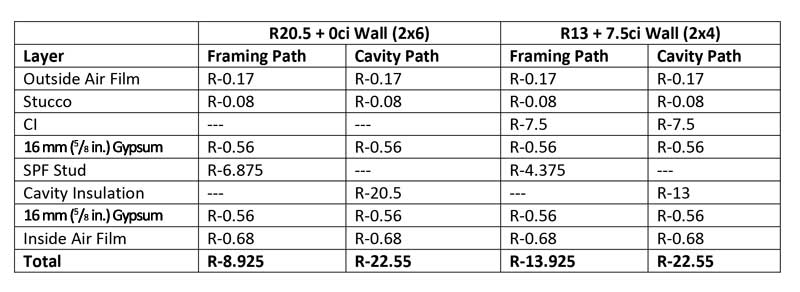# Energy code math for commercial walls: How polyiso continuous insulation can helpFigure 3: A list of the layers for each path heat can take through the walls and their R-values.

Good energy code math
For wood walls, the “parallel path” method is appropriate, (see for example, the “R 20.5 + 0 ci” and “R 13 + 7.5” wall assemblies in Figure 1). This method of calculation can be applied to any combination of ci and cavity insulation required for wood-framed construction (whether commercial or residential). The first step is to determine the R-value for each “path” heat can take through the wall. There are two paths—through the framing (studs and headers) and cavity. In Figure 3, the layers for each path and their R-values are listed. The totals are obtained by summing the R-values for each layer in each path.

One can see while both walls have the same cavity path R-value, the R 13 + 7.5 ci wall has a higher R-value for the framing path (even with a smaller thermal contribution from the thinner 2 x 4 wall framing), thanks to the ci.

The next step is to combine the R-values of the two paths to get an overall value for the entire wall assembly. To do this, the author assumes the wall assemblies are 25 percent framing (21 percent studs and 4 percent headers) and 75 percent cavity by area, which is typical for 406 mm (16 in.) o.c. framing. Then the U-factor for each wall can be obtained with the following formula:

U=ffframing*1/Rframing +ffcavity*1/Rcavity

where ff is the framing factor (25 percent for framing and 75 percent for cavity). Once the U-factor is found, the assembly R-value is just the inverse of the U-factor. This calculation gives us the assembly U-factors and R-values found in
Figure 4.